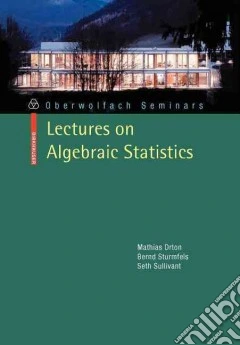ricerca
avanzata

# Lectures on Algebraic Statistics

## Un libro in lingua di Mathias DrtonBernd SturmfelsSeth Sullivant edito da Springer Verlag, 2009

• € 33,40
• Il prezzo è variabile in funzione del cambio della valuta d’origineHow does an algebraic geometer studying secant varieties further the understanding of hypothesis tests in statistics? Why would a statistician working on factor analysis raise open problems about determinantal varieties? Connections of this type are at the heart of the new field of "algebraic statistics". In this field, mathematicians and statisticians come together to solve statistical inference problems using concepts from algebraic geometry as well as related computational and combinatorial techniques. The goal of these lectures is to introduce newcomers from the different camps to algebraic statistics. The introduction will be centered around the following three observations: many important statistical models correspond to algebraic or semi-algebraic sets of parameters; the geometry of these parameter spaces determines the behaviour of widely used statistical inference procedures; computational algebraic geometry can be used to study parameter spaces and other features of statistical models.